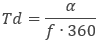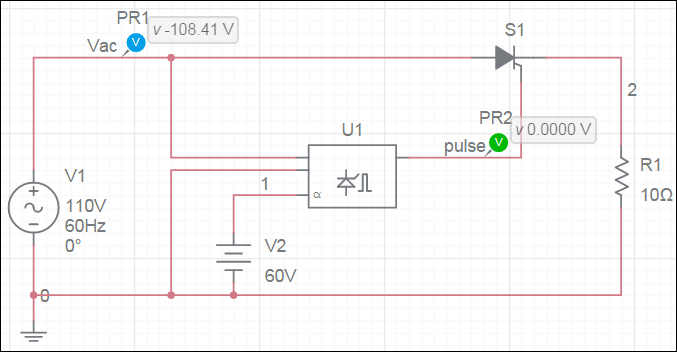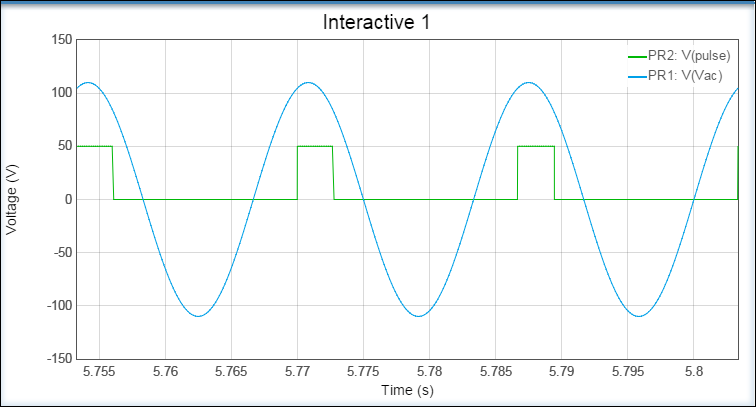OK

# Phase angle controller

This component models a simple phase angle controller for controlling a single thyristor. It generates a voltage pulse on pin T some delay after a rising zero-crossing voltage occurs between pins Ph+ and Ph-. The delay is set using the voltage applied to the firing angle pin, α. The voltage on this pin is assumed to be the delay in degrees.

The actual time delay, in seconds, is calculated as follows:where

f = Frequency parameter
α = firing angle (voltage at pin α)

Example:Parameters:

Frequency - 60 Hz
Pulse_width - 50 degrees
Pulse_amplitude - 50 V

Results: